# Matrix Transpose

The transpose of a matrix is denoted by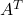is obtained by changing rows into columns or columns to rows of a matrix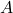. If size of the matrixis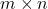then the size of the transposed matrixis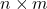.

### Transpose Of A Matrix

The element in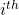row and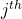column of matrixbecomes therow andcolumn element in matrix.

\begin{aligned}
&(A^T)_{ij} = (A)_{ji}
\end{aligned}

Letbe a matrix of size.

\begin{aligned}
A = \begin{bmatrix}a & b\\ c & d\\ e & f\end{bmatrix}
\end{aligned}

Transpose of matrix.

\begin{aligned}
A^T = \begin{bmatrix}a & c & e\\ b & d & f\end{bmatrix}
\end{aligned}

Let us take element ‘c’ which is at 2nd row and 1st column of matrix; after transpose operation on matrix, it is at the position of 1st row and 2nd column of matrix.

Similarly, the element ‘b’ is at the position of first row and second column of matrix, but after the transpose operation, its position changes to 2nd row and 1st column in matrix.

Example #1

Transpose the following matrix A.

\begin{aligned}
A = \begin{bmatrix}3 & 1 & 5\\ 2 & 6 & 9\end{bmatrix}
\end{aligned}

The transpose of matrixis

\begin{aligned}
A^T = \begin{bmatrix}3 & 2\\ 1 & 6\\5 & 9\end{bmatrix}
\end{aligned}

Example #2

Transpose the following matrix B.

\begin{aligned}
&A = \begin{bmatrix}1 & 5\\ 7 & 6\\8 & 4\end{bmatrix}
\end{aligned}

The transpose of matrixis

\begin{aligned}
A^T = \begin{bmatrix}1 & 7 & 8\\ 5 & 6 & 4\end{bmatrix}
\end{aligned}

### Symmetric Matrix

When the transpose of the matrix is the original matrix itself, then it is called a Symmetric matrix. Supposeis a matrix of size, then the transpose of matrix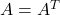.

All the elements above the diagonal is a mirror image of elements below the diagonal elements. That is,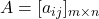is symmetric matrix if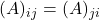for all i and j.

\begin{aligned}
A = \begin{bmatrix}a_{11} & p & q\\p & a_{22} & r\\q & r & a_{33}\end{bmatrix}
\end{aligned}

The elements of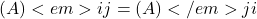. The transpose of such a matrix is,

\begin{aligned}
A^T = \begin{bmatrix}a_{11} & p & q\\p & a_{22} & r\\q & r & a_{33}\end{bmatrix}
\end{aligned}

Therefore,

\begin{aligned}
A = A^T
\end{aligned}

### What Are The Properties Of A Transpose Of A Matrix ?

In this section, we shall discuss about the properties of a transpose of a matrix. There are 4 interesting properties of a transpose as listed below.

1.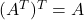, whereis a matrix of sizeor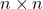.
2.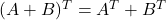, whereandare of same size, that is,or.
3.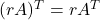, whereis matrix of sizeorand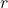is a real number.
4.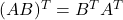, whereandare matrices of size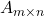and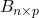.

Let us verify each of the statement.

#### #1 :The transpose of a transpose of matrixis the original matrix.

\begin{aligned}
Let \hspace{5px}A = \begin{bmatrix}2 & 3\\-1 & 5\end{bmatrix}
\end{aligned}

Transpose of matrix.

\begin{aligned}
A^T = \begin{bmatrix}2 & -1\\3 & 5\end{bmatrix}
\end{aligned}

Transpose of.

\begin{aligned}
(A^T)^T = \begin{bmatrix}2 & 3\\-1 & 5\end{bmatrix}
\end{aligned}

From the results above, it is clear thatwhereis a matrix of sizeor.

#### #2 :The transpose of sum of two matricesandof same sizeoris equal to sum of transpose of matricesand.

Letandbe two matrices of same size. Then

\begin{aligned}
&A = \begin{bmatrix}1 & 5\\-2 & 3\end{bmatrix}   B = \begin{bmatrix}2 & 1\\5 & -1\end{bmatrix}\\\\
&(A + B) = \begin{bmatrix}3 & 6\\3 & 2\end{bmatrix}
\end{aligned}

Transpose of matrix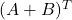.

\begin{aligned}
(A + B)^T = \begin{bmatrix}3 & 3\\6 & 2\end{bmatrix}
\end{aligned}

Now, we shall take transpose of matrixand matrixand add them together to obtain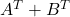.

\begin{aligned}
A = \begin{bmatrix}1 & 5\\-2 & 3\end{bmatrix}   B = \begin{bmatrix}2 & 1\\5 & -1\end{bmatrix}
\end{aligned}

Transpose of A.

\begin{aligned}
A^T = \begin{bmatrix}1 & -2\\5 & 3\end{bmatrix}
\end{aligned}

Transpose of B.

\begin{aligned}
B^T = \begin{bmatrix}2 & 5\\1 & -1\end{bmatrix}
\end{aligned}

Sum ofand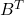.

\begin{aligned}
A^T + B^T = \begin{bmatrix}3 & 3\\6 & 2\end{bmatrix}
\end{aligned}

#### #3 :A transpose of the product of matrixwith scalaris equal to the product of scalarand transpose of matrixwhere size of the matrixisorandis a real number.

\begin{aligned}
&Let \hspace{5px} A = \begin{bmatrix}2 & 3\\1 & 7\end{bmatrix} \hspace{4px} and \hspace{5px} r = 2\\\\
&(rA) = \begin{bmatrix}4 & 6\\2 & 14\end{bmatrix}
\end{aligned}

Transpose of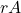,

\begin{aligned}
(rA)^T = \begin{bmatrix}4 & 2\\6 & 14\end{bmatrix}
\end{aligned}

Similarly, let us take transpose of.

\begin{aligned}
A^T = \begin{bmatrix}2 & 1\\3 & 7\end{bmatrix}
\end{aligned}

The product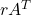is,

\begin{aligned}
rA^T = \begin{bmatrix}4 & 2\\6 & 14\end{bmatrix}
\end{aligned}

Therefore,.

The output of both the products are equal and the propertyis true for all matrices.

#### #4 :The transpose of product of two defined (and) matricesandis equal to the product of transpose of matrixand transpose of matrix. Let us verify this claim with the help of an example.

\begin{aligned}
&Let \hspace{5px}A = \begin{bmatrix}1 & 5\\2 & 1\end{bmatrix} \hspace{5px} and \hspace{5px} B = \begin{bmatrix}3 & -1\\2 & 3\end{bmatrix}\\\\
&AB = \begin{bmatrix}3 + 10 & -1 + 15\\6 + 2 & -2 + 3\end{bmatrix}= \begin{bmatrix}13 & 14\\8 & 1\end{bmatrix}
\end{aligned}

Transpose of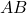.

\begin{aligned}
(AB)^T = \begin{bmatrix}13 & 8\\14 & 1\end{bmatrix}
\end{aligned}

Similarly, the transpose of matrixand matrixis,

\begin{aligned}
&B^T = \begin{bmatrix}3 & 2\\-1 & 3\end{bmatrix} \hspace{5px} and \hspace{5px} A^T = \begin{bmatrix}1 & 2\\5 & 1\end{bmatrix}\\\\
&B^TA^T = \begin{bmatrix}3 + 10 & 6 + 2\\-1 + 15 & -2 + 3\end{bmatrix}\\\\
&B^TA^T = \begin{bmatrix}13 & 8\\14 & 1\end{bmatrix}\\\\
&Therefore, \hspace{5px}(AB)^T = B^TA^T
\end{aligned}

Once again, the product of both sides of the equation of the propertyholds true. The property is valid.

In the next, post we will discuss more about symmetric and skew-symmetric matrices.